In Glattfelder, Dupuis and Olsen (2011) and several previous articles, it has been shown that there is a shortage of information if we only use equidistant physical time, as is conventionally done. They use the tick-by-tick data of the foreign exchange market and ‘event-based’ time rather than physical time. For example, if an asset return is equal to or above a certain value, that value is called the ‘threshold’ and a return that surpasses that value is defined as an ‘event’. Then they analyze movements between those events as they repeat, trying to find a certain scaling law. Time between events is considered as a sum of smaller events, self-defined ‘ticks.’ Throughout their paper, they define tick as 2 basis points (2/100 of a percent) In this paper, however, a tick is defined as 1 basis point since our data has higher frequency. Due to the computational burden, I only could find some laws in a smaller sample.

Scaling Laws

Scaling laws are widely used in other scientific fields including recently in economics and finance. Very generally, they describe relationships between a quantity and its frequency. In Newman (2005), there are many examples of scaling laws in different fields. For example, the length of a word in English is found to be ‘scale-invariant’ to its usage frequency. Another example in Newman (2005) refers to the relation of the number of cities to their population size.

Clearly, taking logarithms of both sides of an equation would yield a linear relationship. For scaling laws in foreign exchange data discovered in Glattfelder, Dupuis and Olsen (2011), instead of frequencies we have the average number of ticks, the average time intervals, and so on. For quantities, we mainly have certain return values and time intervals, which they call ‘thresholds.’ For example, their research relates the average number of ticks (self-defined, they define it as 2 basis points, I define it as 1 basis point) that happen during a return threshold event to that threshold. Taking logarithms yields us a linear relationship and we can pin down parameters via simple OLS.

Data and Methodology

The data we use is liquid stock data of milliseconds. I had a full year (2016) of data but due to limited computing power I use a smaller sample. I use mid-prices of quotes as suggested in Glattfelder, Dupuis and Olsen (2011) and ignore those returns which are not bigger than the defined tick in this paper, i.e. 1 basis point. Our sample comes to around 70,000 points. Even with that I could not extract a scaling law since the code I have written in R takes hours. However, considering the range of the sample and the self-defined tick, there are some preliminary results. For a scaling law, related time intervals of one day are used. Thus, I use 10,000 points of returns price move and directional change relationships and around 120,000 data points for a related time interval law.

Results

The first result gives a scaling law between a range of return thresholds and the average number of ticks. All returns (simple returns are used) that are smaller than 1 basis point (defined tick) are removed and the threshold range is defined between 2 and 8 basis points with 0.1 basis point increments. Only the first 10,000 observations are used due to very low speed in computing power. This method gives us 61 observation points, consisting of 61 return thresholds and average number of ticks (1 basis point moves) each time a threshold event happens. For example, if a return threshold is 3 basis points (0.03%), each time a return of 3 basis points or higher occurs, ticks up to that point and between are calculated and averaged. This procedure needs outer and inner loops and conditions inside loops that cause very low speed. If we take whole data points, we must change the threshold range and increment intervals accordingly to capture a scaling law. Below is a simple graph using pure data points that implies a power relationship.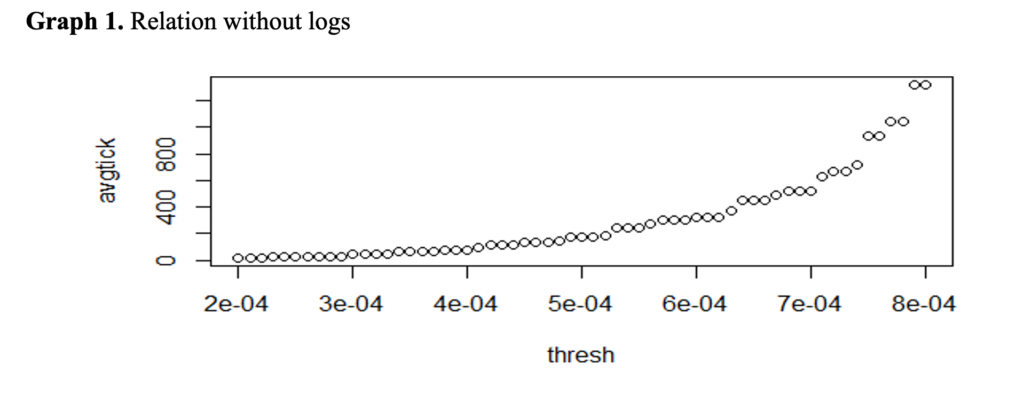The above graph shows a relationship between average tick numbers and return thresholds, in log scales. Apparently, there is a linear relationship visible to the naked eye. We can run a simple OLS regression to get stronger evidence. From regression, we get R2 = 0.9879 and adjusted-R2=0.9877, as expected. Our coefficient estimates are highly significant and we have very low residual standard error (0.1425). I am hesitant to speculate on the quantities of the parameters, since I use a very small sample size. If we used a different sample size or, ideally, many years of tick-by-tick quote data of milliseconds, we could make stronger claims on the ‘universality’ of our parameter values. In general, this law implies that to reach a certain high return (in absolute terms), we need more ‘intrinsic time’ of which the smallest unit is a ‘tick’.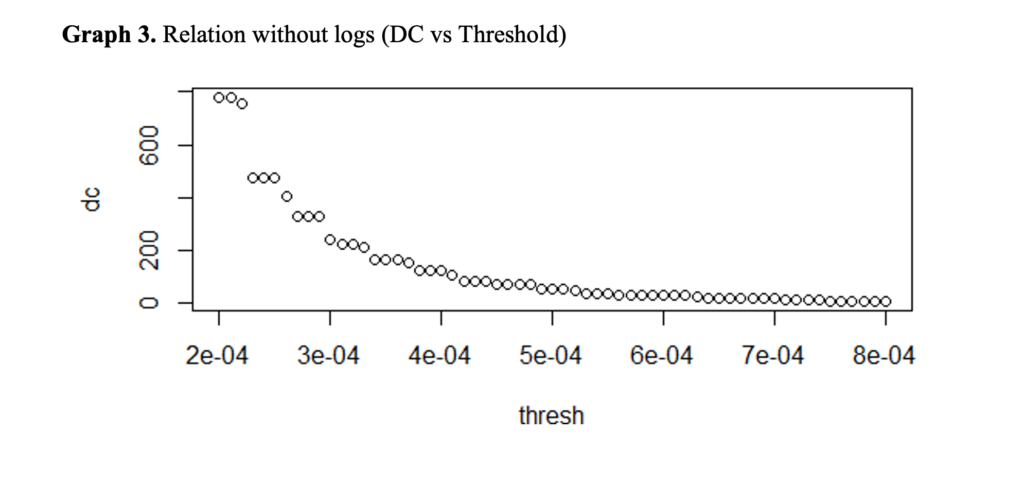The graph above implies a power relation between return thresholds and ‘directional change’ events as they are defined in Glattfelder, Dupuis and Olsen (2011). Given a certain return threshold, let us assume 5 basis points (0.05%), these events are those that are higher than 5 basis points or are lower than negative 5 basis points. Below is a graph in log scales.

##OLS results are identical with the first law derived.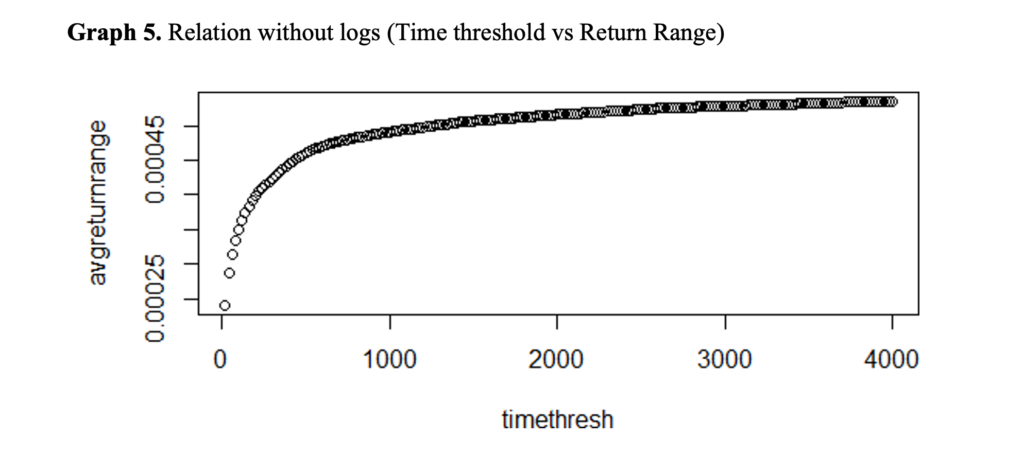Above is the graph showing the average maximal return range for each time threshold. Time thresholds are from 20 seconds to 4,000 seconds, yielding 200 observations. Only one day of data is used. Below is the graph in log scales and OLS results yield high R2.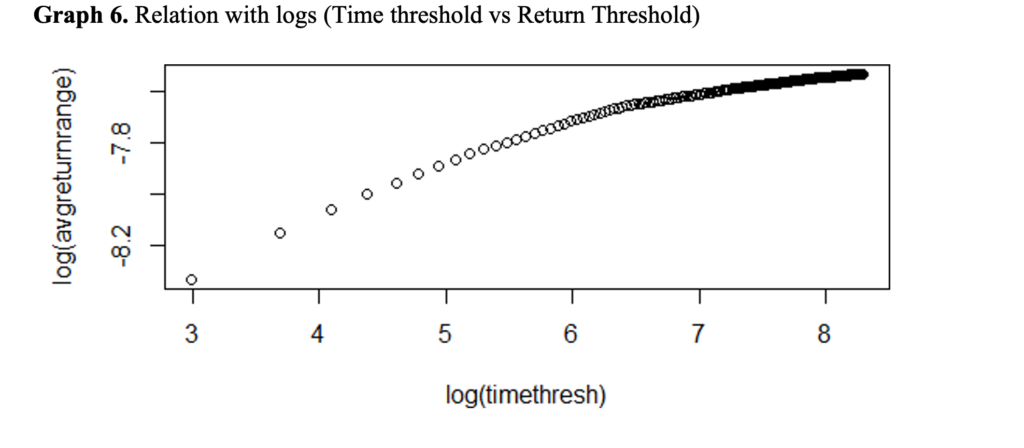In Glattfelder, Dupuis and Olsen (2011), they have a scaling law, however, for average maximal price range, not a return range. Nevertheless, they have graphs showing percentage values. That is why I tried return range first. It appears that both relations might hold. Below are the results of average maximal price range. It might suggest that there is an extra scaling law in the return range as well.

##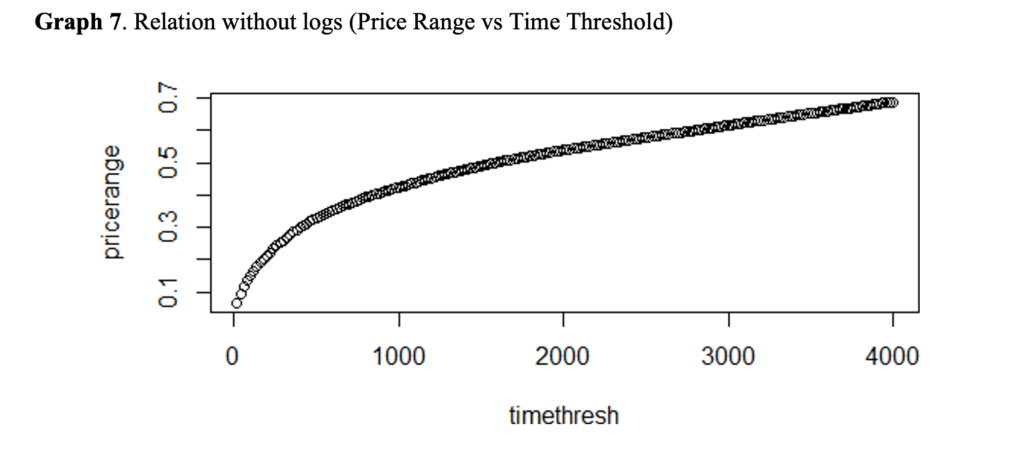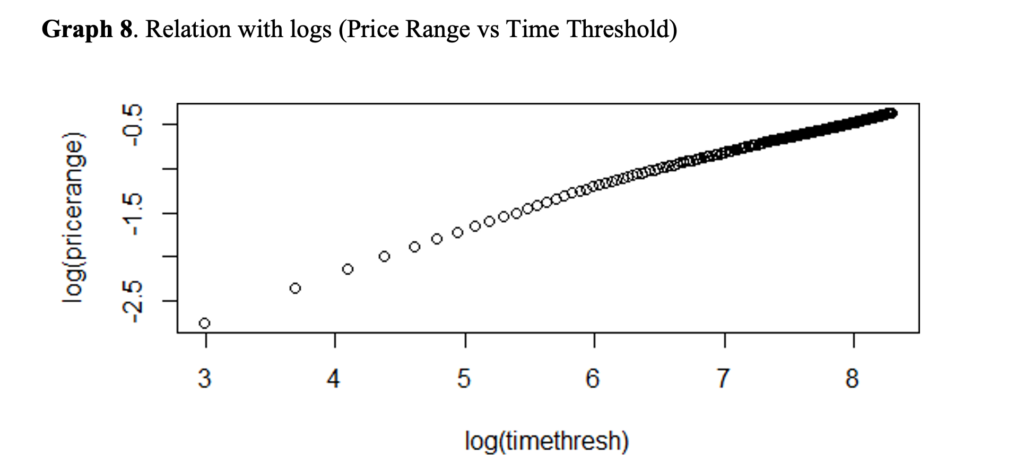Conclusion and Further Discussion

I tried to explore scaling law relationships for stock price data, like those in Glattfelder, Dupuis and Olsen (2011), for foreign exchange data. Using a small data set I was able to extract similar results. However, due to limited computational power and coding problems (we need very detailed algorithms) I could not test everything that was suggested in Glattfelder, Dupuis and Olsen (2011). Many stocks for many years should be researched in detail, in order to make stronger claims. The main outcome of this paper is that it is important to be careful about the range of the variables if we want to capture a scaling law. Another point is that once we go to a higher frequency, there might be different scaling laws, or some of the laws for lower frequency data might not show up. If we had a higher frequency than milliseconds we might have more laws. Moreover, if we had brokers’ data and algorithms, we could explore the reasons why the scaling laws arise. One main shortage of this paper is that I could not figure out an algorithm for extracting ‘overshoot’ relations as done in Glattfelder, Dupuis and Olsen (2011). Future research might include building real trading strategies using scaling laws and testing profit opportunities.

References:

2005. E. J. Newman. Power laws, pareto distributions and zipf’s law. Contemp. Phys., 46 (5):323 – 351, 2005.

Glattfelder, J. B., Dupuis, A., & Olsen, R. B. (2011). Patterns in high-frequency FX data: discovery of 12 empirical scaling laws. Quantitative Finance, 11(4), 599-614.